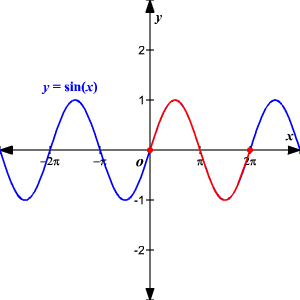# Periodic Functions

Periodic functions are functions that behave in a cyclic (repetitive) manner over a specified interval (called a period).  The graph repeats itself over and over as it is traced from left to right.  In other words, the entire graph can be formed from copies of a particular portion, repeated at regular intervals indefinitely.  If $f$ is known over one period then it is known everywhere.

More formally, a function $f$ is periodic if there exists a real number $P$ such that $f\left(x+P\right)=f\left(x\right)$ for all $x$ .